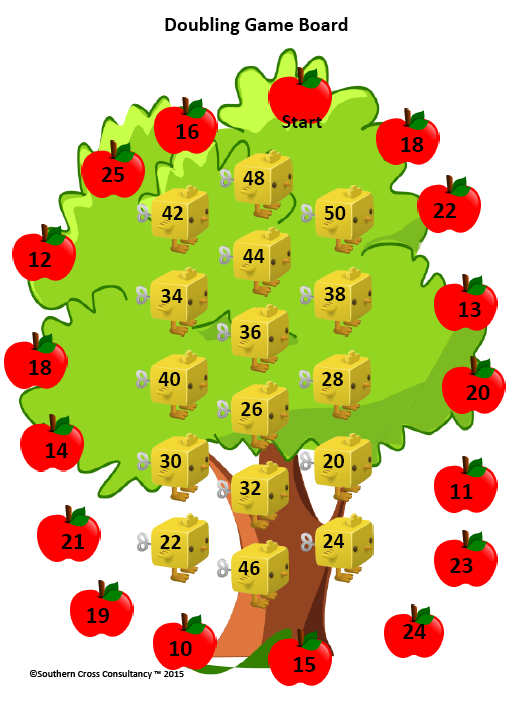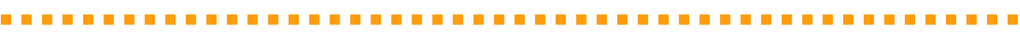Math Resources and Games# Grade 2 Math CCSS Games Packet (over 100+ Games!)

\$14.95

In Grade 2, instructional time should focus on four critical areas:

(1) extending understanding of base-ten notation;

(2) building fluency with addition and subtraction;

(3) using standard units of measure; and

(4) describing and analyzing shapes.

Southern Cross Consultancy have developed over 100 hands-on games, Math centers and activities for use in the classroom utilizing the "I Can" format to reinforce the student-friendly instructions, reinforcing student independence.

The Grade 2 Math CCSS Games Packet includes:OPERATIONS & ALGEBRAIC THINKING
Represent and solve problems involving addition and subtraction
2OA1. Use addition and subtraction within 100 to solve one- and two-step word problems involving situations of adding to, taking from, putting together, taking apart, and comparing, with unknowns in all positions, e.g., by using drawings and equations with a symbol for the unknown number to represent the problem.

Add To Change Unknown to 100*
Add To Result Unknown to 100
Add To Start Unknown to 100*
Change Unknown to 100*
Compare Bigger Unknown Fewer version to 100*
Compare Bigger Unknown More version to 100*
Compare Difference Unknown How Many Fewer version to 100*
Compare Difference Unknown How Many More version to 100*
Compare Smaller Unknown Fewer version to 100*
Compare Smaller Unknown More version to 100*
Join Together Result Unknown to 100*
Put Together Take Apart Addend Unknown to 100*
Put Together Take Apart Both Addends Unknown to 100*
Put Together Take Apart Total Unknown*
Start Unknown Addition Stories to 100*
Take From Change Unknown to 100*
Take From Result Unknown to 100*
Take From Start Unknown to 100*
Two Step Word Problems to 100*

2OA2. Fluently add and subtract within 20 using mental strategies. By end of Grade 2, know from memory all sums of two one-digit numbers.

1 More Than, 1 Less Than Bingo to 20*
Adding 8 with a Ten Frame*
Adding 9 with a Ten Frame*
Addition Bingo Facts to 12 and 20*
Cross Outs on the Number Line*
Double Facts Posters*
Double Trouble
Doubles Match to 20*
Doubles Quest*
Finding More or Less*
Finding More or Less Version 2*
Plus 8*
Plus 9*
Popsicle Stick Subtraction Game*
Roll and Subtract*
Roll and Subtract Version 2*
Subtraction Difference*
The Fair Car Race*
The Number Target Game*
Walk the Dog Domino Game*

Work with equal groups of objects to gain foundations for multiplication
2OA3. Determine whether a group of objects (up to 20) has an odd or even number of members, e.g., by pairing objects or counting them by 2s; write an equation to express an even number as a sum of two equal addends.  Odd + Odd is the Same as*
Odds and Evens
2OA4. Use addition to find the total number of objects arranged in rectangular arrays with up to 5 rows and up to 5 columns; write an equation to express the total as a sum of equal addends.  Multiplication Array
NUMBER AND OPERATIONS IN BASE 10
Understand place value

2NBT1. Understand that the three digits of a three-digit number represent amounts of hundreds, tens, and ones; e.g., 706 equals 7 hundreds, 0 tens, and 6 ones.

2NBT1a. 100 can be thought of as a bundle of ten tens — called a “hundred.”

2NBT1b. The numbers 100, 200, 300, 400, 500, 600, 700, 800, 900 refer to one, two, three, four, five, six, seven, eight, or nine hundreds (and 0 tens and 0 ones).

2NBT2. Count within 1000; skip-count by 5s, 10s, and 100s.

Calculator Count by 5’s
Calculator Count by 10’s
Count Down Set A*
Count Down Set B*
Count Down Set C*
Counting Hearts*
Covered Numbers*
Hundreds Grid Pieces*
Missing Number Puzzles 100*

2NBT3. Read and write numbers to 1000 using base-ten numerals, number names, and expanded form.  How Many Ways to Expand*
Place Value Dominoes*
Rolling, Choosing, Expanding
2NBT4. Compare two three-digit numbers based on meanings of the hundreds, tens, and ones digits, using >, =, and < symbols to record the results of comparisons.  Flash*
Rolling and Choosing*
Rolling, Choosing and Expanding
Spin and Win*
Use place value understanding and properties of operations to add and subtract
2NBT5. Fluently add and subtract within 100 using strategies based on place value, properties of operations, and/or the relationship between addition and subtraction. 75 Popsicle Stick Game
Between Two Numbers
Doubles*
Friendly Numbers*
Friendly Numbers One Digit +5, +10*
Friendly Numbers Two Digit +5, +10, +20*
Friendly Numbers Two Digit +5, +10, +20,+50*
Missing Numbers 2 Digits*
Partners to 100*
Race to Zero and Math Journal*
Spin and Add Version 1 (no regrouping)*
Spin and Add Version 2 (regrouping)*
from 100*
2NBT6. Add up to four two-digit numbers using strategies based on place value and properties of operations.  Breaking Each Number Into Its Place Value to Add (2 addends, no regrouping)*
Breaking Each Number Into Its Place Value to Add (2 addends, regrouping)*
Breaking Each Number Into Its Place Value to Add (3 addends, no regrouping)*
Breaking Each Number Into Its Place Value to Add (3 addends, regrouping)*
Breaking Each Number Into Its Place Value to Add (4 addends, no regrouping)*
Breaking Each Number Into Its Place Value to Add (4 addends, regrouping)*
2NBT7. Add and subtract within 1000, using concrete models or drawings and strategies based on place value, properties of operations, and/or the relationship between addition and subtraction; relate the strategy to a written method. Understand that in adding or subtracting three-digit numbers, one adds or subtracts hundreds and hundreds, tens and tens, ones and ones; and sometimes it is necessary to compose or decompose tens or hundreds.  Closest to 500
Friendly Numbers Three Digit +10, +20, +50, +100*
How Many to Get to 100 - multiples of 10*
How Many to Get to 100?*
How Many to Get to 200?*
How Many to Get to 300?*
How Many to Get to 600?*
How Many to Get to 1000?*
Partial Sum *
2NBT8. Mentally add 10 or 100 to a given number 100–900, and mentally subtract 10 or 100 from a given number 100–900. Jump Back to 0
Listen In Cards*
2NBT9. Explain why addition and subtraction strategies work, using place value and the properties of operations.  Explaining How I Know My Strategy Works
Explaining My Strategies*
MEASUREMENT AND DATA
Measure and estimate lengths in standard units
2MD1. Measure the length of an object by selecting and using appropriate tools such as rulers, yardsticks, meter sticks, and measuring tapes.

2MD2. Measure the length of an object twice, using length units of different lengths for the two measurements; describe how the two measurements relate to the size of the unit chosen.  Measuring in Inches and Centimeters
2MD3. Estimate lengths using units of inches, feet, centimeters, and meters.  Estimating and Measuring – Centimeters*
Estimating and Measuring – Feet*
Estimating and Measuring – Inches*
Estimating and Measuring – Meters*
Finding Foot Measurements
Finding Meter Measurements
2MD4. Measure to determine how much longer one object is than another, expressing the length difference in terms of a standard length unit. Comparing Measurements – Centimeters
Comparing Measurements – Inches
Relate addition and subtraction to length
2MD5. Use addition and subtraction within 100 to solve word problems involving lengths that are given in the same units, e.g., by using drawings (such as drawings of rulers) and equations with a symbol for the unknown number to represent the problem.  Addition and Subtraction Length Word Problems - one step
Addition and Subtraction Length Word Problems - two step*
2MD6. Represent whole numbers as lengths from 0 on a number line diagram with equally spaced points corresponding to the numbers 0, 1, 2, …, and represent whole-number sums and differences within 100 on a number line diagram.  Number Line Riddles
Work with time and money
2MD7. Tell and write time from analogue and digital clocks to the nearest five minutes, using a.m. and p.m.  Time Bump Set A*
Time Bump Set B*
Time is of the Essence*
Time Matching Game
2MD8. Solve word problems involving dollar bills, quarters, dimes, nickels, and pennies, using \$ and ¢ symbols appropriately. Example: If you have 2 dimes and 3 pennies, how many cents do you have?  Money Word Problems Set A*
2M8 Money Word Problems Set B*
Money Word Problems Set C*
Money Word Problems Set D*
Race to a Dollar
Represent and interpret data
2MD9. Generate measurement data by measuring lengths of several objects to the nearest whole unit, or by making repeated measurements of the same object. Show the measurements by making a line plot, where the horizontal scale is marked off in whole-number units.
2MD10. Draw a picture graph and a bar graph (with single-unit scale) to represent a data set with up to four categories. Solve simple put-together, take-apart, and compare problems 1 using information presented in a bar graph.
GEOMETRY
Reason with shapes and their attributes
2G1. Recognize and draw shapes having specified attributes, such as a given number of angles or a given number of equal faces.1 Identify triangles, quadrilaterals, pentagons, hexagons, and cubes.  Describing Plane Shapes
Geoboard Shapes*
2G2. Partition a rectangle into rows and columns of same-size squares and count to find the total number of them.
2G3. Partition circles and rectangles into two, three, or four equal shares, describe the shares using the words halves, thirds, half of, a third of, etc., and describe the whole as two halves, three thirds, four fourths. Recognize that equal shares of identical wholes need not have the same shape.